# Combine Duplicate Rows and Sum the Values

This post will teach you how to combine duplicate rows and sum the corresponding values or calculate numbers in specific column in excel. And how to merge duplicate rows and then sum the values with VBA macro in Excel. How to combine rows with same name and sum the value.

You can use the Consolidate feature to combine duplicate rows and then sum the values in excel, let’s see the below steps:

1# select a cell that you want to display the result combined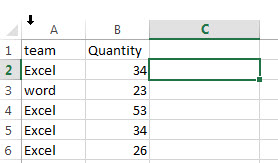2# on the DATA tab, click Consolidate command under Data Tools group.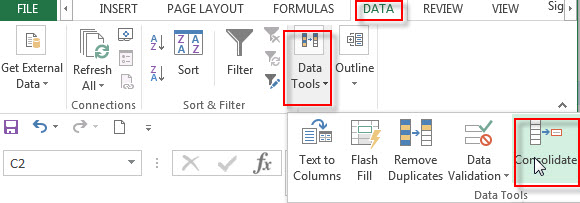3# the Consolidate window will appear.

4# choose Sum from Function: drop-down list, select the range that you want to combine, then click Add button to add it in the All references box. Select Top row and Left column checkbox. Then click OK button.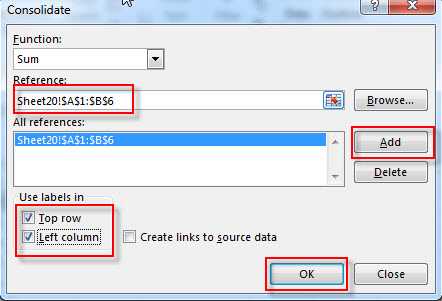5# you will see that all duplicate rows are combined and the corresponding values summed.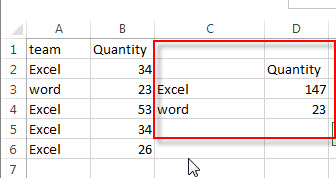## Combine Duplicate Rows and Sum the Values with VBA code

You can also combine duplicate rows and sum the values with VBA code in Excel. Just do the following:

1# click on “Visual Basic” command under DEVELOPER Tab.2# then the “Visual Basic Editor” window will appear.

3# click “Insert” ->”Module” to create a new module4# paste the below VBA code into the code window. Then clicking “Save” button.

```Sub CombineDuplicateRowsAndSum()
Set R = Application.Selection
Set R = Application.InputBox("select one Range:", "CombineDuplicateRowsAndSum", R.Address, Type:=8)
Set Dic = CreateObject("Scripting.Dictionary")
arr = R.Value
For i = 1 To UBound(arr, 1)
Dic(arr(i, 1)) = Dic(arr(i, 1)) + arr(i, 2)
Next
R.ClearContents
R.Range("A1").Resize(Dic.Count, 1) = Application.WorksheetFunction.Transpose(Dic.keys)
R.Range("B1").Resize(Dic.Count, 1) = Application.WorksheetFunction.Transpose(Dic.items)
Application.ScreenUpdating = True
End Sub```

5# back to the current worksheet, then run the above excel macro.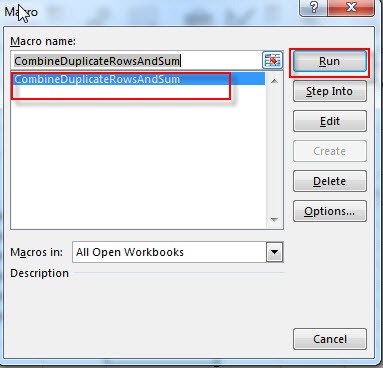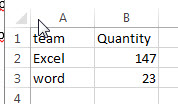Related Posts

Excel/Google Sheets: Full Row Reference

Excel or Google sheets supports both full row references and full column references. This article will talk about the usage of full row references. Full Row reference is another cell reference in Excel or Google Sheets, which is used to ...

Excel/Google Sheets: Full Column Reference

Full column reference is another cell reference in Excel or Google Sheets, which is used to reference the entire column, for example, if you want to reference the entire column A, you can use the following reference format A: A. ...

Excel/Google Sheets: Expanding Reference

An expanding reference is a reference type in Excel and Google worksheets that extends the range of a cell when a formula is copied to the cell below or to the right. In this article, we will explain how to ...

Excel/Google Sheets: Mixed Reference

In Microsoft Excel Spreadsheet or Google sheets, there is another cell reference, mixed references, where part of the reference is absolute, part of the relative. This article will describe how to use mixed references through specific examples. Mixed Reference When ...

Excel/Google Sheets: Absolute Reference

In Microsoft Excel spreadsheet or Google Sheets, the cell reference is the cell or cell area address or name in the worksheet, the cell reference contains two types of references, namely, relative references and absolute references. Absolute Reference An absolute ...

Sidebar